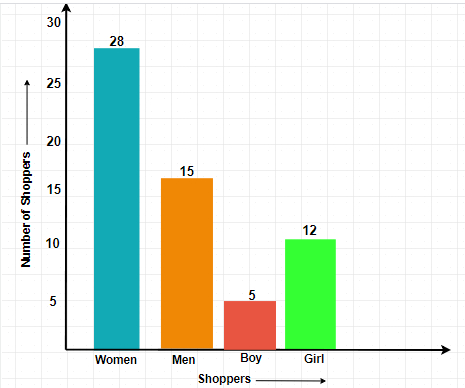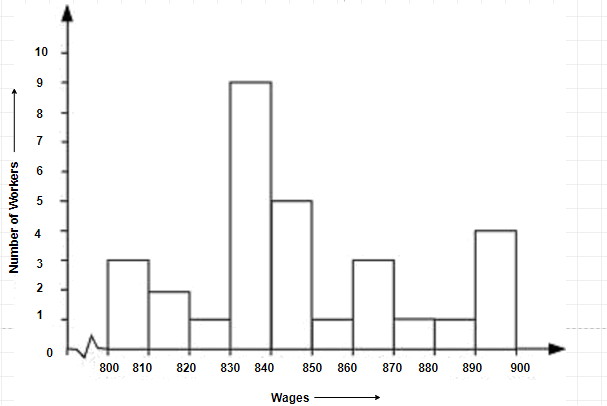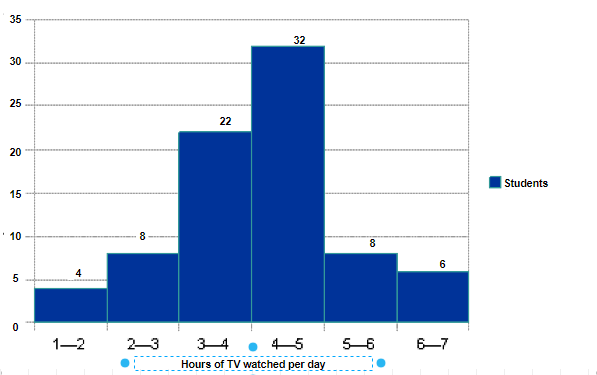# Excercise 5.1 Data Handling- NCERT Solutions Class 8

Go back to  'Data Handling'

## Chapter 5 Ex.5.1 Question 1

For which of these would you use a histogram to show the data:

(a) The number of letters for different areas in a postman’s bag.

(b) The height of competitors in athletics meet.

(c) The number of cassettes produced by $$5$$ companies.

(d) The number of passengers boarding trains from $$7.00$$ a.m. to $$7.00$$ p.m. at a station.

Give reason for each.

### Solution

What is known?

$$4$$ different conditions.

What is unknown?

The statement that will use histogram to show the data.

Reasoning:

Histogram is a graphical representation of data, if data represented in a manner of class- interval.

Steps:

Since, Histogram is a graphical representation of data, the data is represented in manner of class- interval.

In case (i), The number of areas cannot be represented in class interval. So, we cannot use the histogram to show the data.

In case (ii), Height of competitors can be divided into intervals. So, we can use histogram here.

For example,

 Height in (cm) No. of competitors $$150-160$$ $$160-170$$ $$170-180$$ $$180-190$$ $$10$$ $$12$$ $$5$$ $$2$$

In case (iii), Companies cannot be divided into intervals. So, we cannot use histogram here.

In case (iv), Time for boarding the train can be divided into intervals. So, we can use histogram here.

For example,

 Time in hours No. of passengers $$7$$ am – $$10$$ am $$10$$ am – $$1$$ pm $$1$$ pm – $$4$$ pm $$4$$ pm – $$7$$ pm $$1500$$ $$2000$$ $$1000$$ $$800$$

## Chapter 5 Ex.5.1 Question 2

The shoppers who come to a departmental store are marked as: man (M), woman (W), boy (B) or girl (G). The following list gives the shoppers who came during the first hour in the morning.

W W W G B W W M G G M M W W W W G B M W B G G M W W M M W W W M W B W G M W W W W G W M M W W M W G W M G W M M B G G W.

Make a frequency distribution table using tally marks.Draw a bar graph to illustrate it.

### Solution

What is known?

List of the shoppers who came during first hour in the morning.

What is unknown?

Frequency distribution of the shoppers list and bar graph representation.

Reasoning:

Number of occurrences of the particular entry is known as frequency.

Steps:

The frequency distribution table is as follows:

 Shopper Tally Marks Number of Shoppers $$W$$ \\\\ \\\\ \\\\ \\\\ \\\\ \\\ $$28$$ $$M$$ \\\\ \\\\ \\\\ $$15$$ $$B$$ \\\\ $$5$$ $$G$$ \\\\ \\\\ \\ $$12$$ Total $$60$$

The illustration of data by bar-graph is as follows:## Chapter 5 Ex.5.1 Question 3

The weekly wages (in $$\rm{Rs}$$) of $$30$$ workers in a factory are:

$$830,\; 835,\; 890,\; 810,\; 835,\; 836,\; 869,\; 845,\; 898,\; 890,\; 820,\; 860,\; 832,\; 833,\; 855,\; 845,\; 804,\; 808,\; 812,\; 840,\; 885,\; 835,\; 835,\; 836,\; 878,\; 840,\; 868,\; 890,\; 806,\; 840.$$

Using tally marks, make a frequency table with intervals as $$800 – 810,\; 810 – 820$$ and so on.

### Solution

What is known?

Weekly wages of $$30$$ workers in Rupees.

What is unknown?

Frequency table of the weekly wages of $$30$$ workers with intervals of $$10.$$

Reasoning:

Number of occurrences of the particular entry is known as frequency.

Steps:

The representation of data by frequency distribution table using tally marks is as follows:

 Class Intervals Tally Marks Frequency $$800\;–\;810$$ ||| $$3$$ $$810\;–\;820$$ || $$2$$ $$820\;–\;830$$ | $$1$$ $$830\;–\;840$$ |||| |||| $$9$$ $$840\;–\;850$$ |||| $$5$$ $$850\;–\;860$$ | $$1$$ $$860\;–\;870$$ ||| $$3$$ $$870\;–\;880$$ | $$1$$ $$880\;–\;890$$ | $$1$$ $$890\;–\;900$$ |||| $$4$$ Total 30

## Chapter 5 Ex.5.1 Question 4

Draw a histogram for the frequency table made for the data in Question $$3$$ and answer the following questions.

(i) How many workers earn $$\rm{Rs}\; 850$$ and more?

(ii) How many workers earn less than $$\rm{Rs}\;850$$?

### Solution

What is known?

Weekly wages of $$30$$ workers in Rupees.

What is unknown?

1.  Number of workers earning $$850$$ or more.

2.  Number of workers earning less than $$850.$$

Reasoning:

Number of occurrences of the particular entry is known as frequency.

Steps:

(i) $$830\,– \,840$$ group has the maximum number of workers.

(ii) $$10$$ workers can earn more than $$\rm{Rs}\;850.$$

(iii) $$20$$ workers earn less than $$\rm{Rs}\;850.$$## Chapter 5 Ex.5.1 Question 5

The number of hours for which students of a particular class watched television during holidays is shown through the given graph. We draw the histogram for above frequency table:(i) For how many hours did the maximum number of students watch T.V.?

(ii) How many students watched TV for less than $$4$$ hours?

(iii) How many students spent more than $$5$$ hours in watching TV?

### Solution

What is known?

Histogram representing the number of students versus hours of TV watched per day.

What is unknown?

(i) Number of hours did the maximum number of students watch T.V.

(ii) Number of students watched TV for less than $$4$$ hours.

(iii)Number of students spent more than $$5$$ hours in watching TV.

Reasoning:

Histogram is a graphical representation of data, the data represented in manner of class- interval.

Steps:

(i) The maximum number of students watched T.V. for $$4 – 5$$ hours.

(ii) $$34$$ students watched T.V. for less than $$4$$ hours.

(iii) $$14$$ students spent more than $$5$$ hours in watching T.V.

Related Sections
Related Sections
Instant doubt clearing with Cuemath Advanced Math Program